2021 NFL Comeback Player of the Year Odds
-300
0.33 to 1Dak Prescott
50.8% implied probability

+700
7 to 1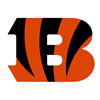Joe Burrow
8.5% implied probability

+1800
18 to 1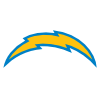Derwin James
3.6% implied probability

+1800
18 to 1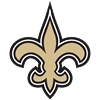Jameis Winston
3.6% implied probability

+2000
20 to 1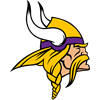Danielle Hunter
3.2% implied probability

+2500
25 to 1Michael Thomas
2.6% implied probability

No changes have been recorded yet.
+2500
25 to 1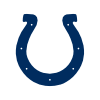Carson Wentz
2.6% implied probability

+4000
40 to 1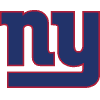Saquon Barkley
1.7% implied probability

+5000
50 to 1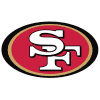Jimmy Garoppolo
1.3% implied probability

+5000
50 to 1Chandler Jones
1.3% implied probability

No changes have been recorded yet.
+5000
50 to 1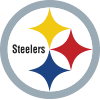Devin Bush
1.3% implied probability

+5000
50 to 1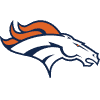Courtland Sutton
1.3% implied probability

+5000
50 to 1George Kittle
1.3% implied probability

+5000
50 to 1O.J. Howard
1.3% implied probability

+5000
50 to 1James Conner
1.3% implied probability

+5000
50 to 1Anthony Barr
1.3% implied probability

+5000
50 to 1Melvin Ingram
1.3% implied probability

+5000
50 to 1Richard Sherman
1.3% implied probability

+5000
50 to 1Von Miller
1.3% implied probability

+5000
50 to 1Nick Bosa
1.3% implied probability

+5000
50 to 1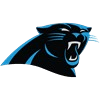Sam Darnold
1.3% implied probability

+5000
50 to 1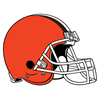Odell Beckham
1.3% implied probability

+5000
50 to 1Christian McCaffrey
1.3% implied probability

+5000
50 to 1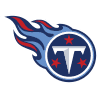Julio Jones
1.3% implied probability

+6000
60 to 1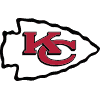Laurent Duvernay-Tardif
1.1% implied probability

+6000
60 to 1Leighton Vander Esch
1.1% implied probability